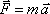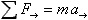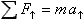Newton's Second Law

Note: This is the big one. One should elaborate on reading the equation and read it as: "The net external force acting on a point particle is equal to the mass of that particle times the acceleration of that particle."

The net external force acting on an object is the vector sum of all the external forces acting on the object. To use Newton's Second Law, one has to first draw a free body diagram of the object (and, if necessary, find the components of vectors that are not along one of the axes of a chosen Cartesian coordinate axes and draw a new free body diagram where such vectors are replaced by their components). Then one uses the free-body diagram to set the sum of the forces along each of the coordinate axes in turn equal to the component of the acceleration directed along that coordinate axis:andTip: This is a cause equals effect equation. The cause on the left equals the effect on the right. When you use this equation, after first writing it down, you should enter into substitution mode. On the left, substitute the applicable forces added together; on the right, substitute the mass times the acceleration. Keep the cause on the left and the effect on the right until you have boiled it down to an algebra problem which you can then solve for the sought quantity.